## Finding dominant colors in an image

Here's a simple task - given an image find the dominant colors in the image. I'll walk you through a lesser known technique that does not use kmeans.

## Implemention in OpenCV

Series: Finding dominant colors in an image:

1. Eigenvectors
2. Implemention in OpenCV
3. Additional functions to view what's happening

I hope the theory covered in the previous part made sense. In this part, we'll implement the technique using OpenCV.

### Setting up the OpenCV project

Let's start by creating a new file, filling in the include files and creating a main function:

```#include <stdio.h>
#include <opencv2/opencv.hpp>
#include <queue>

using namespace std;

int main(int argc, char* argv[]) {
}
```

Let's start by reading some command line arguments. We want the command line to look something like `<command> <image> <color-count>`. Thus the image file would show up in `argv` and the color count parameter would should up in `argv`. We want to ensure the image can be opened and the color count is a number between 1-255.

```int main(int argc, char* argv[]) {
if(argc<3) {
printf("Usage: %s <image> <count>\n", argv);
return 0;
}

char* filename = argv;
cv::Mat matImage = cv::imread(filename);

if(!matImage.data) {
printf("Unable to open the file: %s\n", filename);
return 1;
}

int count = atoi(argv);
if(count <=0 || count >255) {
printf("The color count needs to be between 1-255. You picked: %d\n", count);
return 2;
}

find_dominant_colors(matImage, count);

return 0;
}
```

Once we've done everything, we call the key function of this tutorial - `find_dominant_colors`. Given an image and a color count, it produces a bunch of outputs you can use.

## Finding dominant colors

Before we actually start finding the main colors, let's first design the struct used for storing a tree node.

```...
#include <queue>

using namespace std;

typedef struct t_color_node {
cv::Mat     mean;
cv::Mat     cov;
uchar       classid;

t_color_node *left;
t_color_node *right;
} t_color_node;

int main(int argc, char* argv[]) {
...
```

This structure stores a single tree node. Each node has an associated mean, covariance, class ID and of course the left/right children. We'll work with this struct extensively! Let's define the `find_dominant_color` function now.

```...
} t_color_node;

std::vector<cv::Vec3b> find_dominant_colors(cv::Mat img, int count) {
const int width = img.cols;
const int height = img.rows;

cv::Mat classes = cv::Mat(height, width, CV_8UC1, cv::Scalar(1));
```

The function signature should be self explanatory - given an image and a count, it returns a vector of the dominant colors. We start out by storing the image size in the converience variables `width` and `height`. We also define a `classes` matrix - it is the same size as the original image but specifies which node each pixel belongs to. To start out, we set each pixel to belong to class 1.

```    t_color_node *root = new t_color_node();
root->classid = 1;
root->left = NULL;
root->right = NULL;

t_color_node *next = root;
get_class_mean_cov(img, classes, root);
```

This section of code is about creating the "initial" tree. It currently contains only a single node - the one at the center. No left/right children just yet and it has a class ID of 1. I also created a variable called `next` which we'll use soon. I defined another function called `get_class_mean_cov` - given an image, the class each pixel belongs to and the node we're interested in (class ID of 1, in this case), it calculates the mean and statistics of those pixels. This is used to kick off the entire process.

```    for(int i=0;i<count-1;i++) {
next = get_max_eigenvalue_node(root);
partition_class(img, classes, get_next_classid(root), next);
get_class_mean_cov(img, classes, next->left);
get_class_mean_cov(img, classes, next->right);
}
```

Okay - that was quite a lot of functions. Let me explain what each does.

The `for` loop is used to perform subsequent splits. For n colors, we need to perform n-1 splits in the tree - thus we limit the `for` loop to `count-1`.

The `get_max_eigenvalue_node` function is used to find the leaf node with the largest eigenvalue. This will be done by using a breadth first search (as you'll see later on).

This node corresponds to the color that has the maximum variance - thus it probably contains two colors instead of one. We discussed why we pick the highest eigenvalue in the previous part.

The next function is `partition_class` updates the `classes` matrix. Given the class to partition (`next` in this case) and the per pixel classes, it assigns new pixel classes based on eigenvector calculations. The function `get_next_classid` returns the next available class ID. It walks through the tree and finds the maximum class ID on the tree, adds 1 to it and returns it.

You're already familiar with `get_class_mean_cov` - the partition function created two new classes and we need to calculate the statistics on those regions. This is where that happens.

This is where all computation happens. We just need to fill out the different functions and we'll be ready to go! Let's wrap up our current function first, though!

```    std::vector<cv::Vec3b> colors = get_dominant_colors(img, classes, root);

cv::Mat quantized = get_quantized_image(classes, root);
cv::Mat viewable = get_viewable_image(classes);
cv::Mat dom = get_dominant_palette(colors);

cv::imwrite("./classification.png", viewable);
cv::imwrite("./quantized.png", quantized);
cv::imwrite("./palette.png", dom);

return colors;
}
```

The `get_dominant_colors` function just nicely returns an `std::vector<cv::Vec3b>` object. It's just converient.

Finally, I've created three functions to help you visualize what's going on: `get_quantized_image`, `get_viewable_image` and `get_dominant_palette`. These are the images I used in the previous part. And finally we returns the colors vector. And that wraps up our primary function in this tutorial!

## Statistical functions for detecting primary colors

Let's start out by working on the main functions of our technique. We need to work on three function.

### Calculating the mean and covariance of each class

This function's job is to fill in the `mean` and `cov` objects in our tree of `t_color_node`. Given a specific node (with a specific class ID), walk through all pixels and if a pixel belongs to this `t_color_node` (has the same class ID), update the mean and covariance. This is actually really straight forward.

```void get_class_mean_cov(cv::Mat img, cv::Mat classes, t_color_node *node) {
const int width = img.cols;
const int height = img.rows;
const uchar classid = node->classid;

cv::Mat mean = cv::Mat(3, 1, CV_64FC1, cv::Scalar(0));
cv::Mat cov  = cv::Mat(3, 3, CV_64FC1, cv::Scalar(0));
```

We start out by creating some converience variables (`width`, `height` and `classid`). Then we create the matrices that will actually hold our mean and covariances. These are defined as a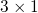and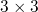matrix because we're working with RGB data.

```    double pixcount = 0;
for(int y=0;y<height;y++) {
cv::Vec3b *ptr = img.ptr<cv::Vec3b>(y);
uchar* ptrClass = classes.ptr<uchar>(y);
for(int x=0;x<width;x++) {
if(ptrClass[x] != classid)
continue;
```

Here, we start looping through all pixels. We use the standard technique of having a pointer per row and then using that pointer to iterate through the columns. We also check if the class at the current pixel matches the class of the `t_color_node` passed in - if not, we're not interested in this pixel (`continue`).

```            cv::Vec3b color = ptr[x];
cv::Mat scaled = cv::Mat(3, 1, CV_64FC1, cv::Scalar(0));
scaled.at<double>(0) = color/255.0f;
scaled.at<double>(1) = color/255.0f;
scaled.at<double>(2) = color/255.0f;

mean = mean + scaled;
cov  = cov + (scaled * scaled.t())
pixcount++
}
}
```

This section of code is where all the statistics happens. Store the color at this pixel into `color` - but this is based on the 0-255 range. Since we're potentially walking over millions of pixels, it's a good idea to scale this down to the 0-1 range to keep things manageable - otherwise you'd run into overflows.

We scale the values from 0-255 to 0-1 to avoid potential overflows. We'll be working on millions of pixels and might run into the limits. Converting to 0-1 doesn't prevent overflows - but gives us more time until we hit the overflow.

To do this, I create a `cv::Mat` to store the RGB of a single pixel in the `CV_64FC1` format and set the three values in this matrix. I use this matrix to update `mean` and `cov` based on the formulae in the previous part. Increment the pixel counter.

```    cov = cov - (mean * mean.t()) / pixcount;
mean = mean / pixcount;

node->mean = mean.clone();
node->cov = cov.clone();
return;
}
```

We had been calculating a partial covariance until now - subtract the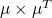matrix to make it complete. Also, the `mean` until now was just a summation - we want to divide it by the number of pixels. Finally, put these values into the `t_color_node` object and return back.

### Find the node with the maximum eigenvalue

Now that we have a function to calculate the mean and covariance for a given class, let's write a function that iterates through the all nodes in our tree and returns a pointer to the node that matches our criteria for partitioning - the class with the highest covariance eigenvalue.

```t_color_node* get_max_eigenvalue_node(t_color_node *current) {
double max_eigen = -1;
cv::Mat eigenvalues, eigenvectors;
std::queue<t_color_node*> queue;
queue.push(current);
```

We start out the function with a variable to keep track of the maximum eigenvalue, two temporary matrices to store the eigenvalues and eigenvectors, a queue (we're doing a BFS traversal). We push the given node to the queue to kickstart the function.

```    t_color_node *ret = current;
if(!current->left && !current->right)
return current;
```

We handle the special case where the provided node has no left or right child. If this is the case, this node has the maximum eigenvalue by default.

```    while(queue.size() > 0) {
t_color_node *node = queue.front();
queue.pop()

if(node->left && node->right) {
queue.push(node->left);
queue.push(node->right);
continue;
}
```

Now we start the BFS. We fetch the item at the front of the queue and remove it. If it has both the left and right pointers set, this isn't a leaf node. We don't need to calculate the eigenvalue here - so we just push the left and right values to the queue and continue. In fact, we'll never run into a case where only the left or only the right node is set - this is because the partition function will always split the node into two.

```        cv::eigen(node->cov, eigenvalues, eigenvectors);
double val = eigenvalues.at<double>(0);
if(val > max_eigen) {
max_eigen = val;
ret = node;
}
}

return ret;
}
```

If a node doesn't have the left and right pointers set, it's a leaf node. So we need to calculate the eigenvalues of the covariance matrix here. The function `cv::eigen` is designed such that it returns the eigenvalues in descending order - so we pick the first eigenvalue. If this is greater than `max_eigen`, we found a new candidate to return.

### Partitioning a class into two

We can calculate the mean / covariance, find the class with the maximum eigenvalue - now we need to split it up. Let's do this!

```void partition_class(cv::Mat img, cv::Mat classes, uchar nextid, t_color_node *node) {
const int width = img.cols;
const int height = img.rows;
const int classid = node->classid;

const uchar newidleft = nextid;
const uchar newidright = nextid + 1;
```

This function will split the given node - setting the left and right pointers. `nextid` is the numeric class ID to use for the newly created class (we use `nextid` for left and `nextid + 1` for right). We also setup some convenience variables like width, height and the class ID of the provided node.

```    cv::Mat mean = node->mean;
cv::Mat cov = node->cov;
cv::Mat eigenvalues, eigenvectors;
cv::eigen(cov, eigenvalues, eigenvectors);

cv::Mat eig = eigenvectors.row(0);
cv::Mat comparison_value = eig * mean;
```

We create a variable for the mean and covairnace of this node. We fetch the first eigenvector (the eigenvector corresponding to the maximum eigenvalue) and use that to calculate `comparison_value` -which is essentially like a threshold. It holds the value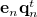from the previous part (look at the section called How do we split?).

The `*` operator acts as a dot product because `eig` is a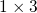matrix and `mean` is amatrix.

```    node->left = new t_color_node();
node->right = new t_color_node();
node->left->classid = newidleft;
node->right->classid = newidright;
```

We create the two new class nodes and set the appropriate class IDs.

```    for(int y=0;y<height;y++) {
cv::Vec3b *ptr = img.ptr<cv::Vec3b>(y);
uchar *ptrClass = classes.ptr<uchar>(y);
for(int x=0;x<width;x++) {
if(ptrClass[x] != classid)
continue;
```

We start iterating through the image pixels. `img` points to the RGB data while `ptrClass` points to the class each pixel belongs to. If the current pixel does not belong to the node we're working on (the `if` condition), we continue onto the next pixel.

```            cv::Vec3b color = ptr[x];
cv::Mat scaled = cv::Mat(3, 1, V_64FC1, cv::Scalar(0));
scaled.at<double>(0) = color/255.0f;
scaled.at<double>(1) = color/255.0f;
scaled.at<double>(2) = color/255.0f;
```

If the pixel does belong to this class, we fetch the RGB value at this pixel and convert it into the 0-1 range (remember our mean and covariances were based on that range?).

```            cv::Mat this_value = eig*scaled;
if(this_value.at<double>(0, 0) <= comparison_value.at<double>(0, 0)) {
ptrClass[x] = newidleft;
} else {
ptrClass[x] = newidright;
}
}
}
return;
}
```

This is where we do the actual split. We check if the dot product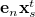is less than or equal to the comparison value. If yes, it belongs to the left node otherwise to the right. The choice of left/right is arbitrary and does not matter.

You've written quite a bit of code until now - we're almost done with the key ideas of this algorithm. Just a few more short functions and we will be able to execute all of this code.

## Wrap up

We have most of the core algorithm done - now we just need to write some additional functions to make our function run. We'll do that in the next part.

### Source code

You can get the source code for this tutorial on Github.

### References

1. M Orchard and C Bouman, Color Quantization of Images, Transactions on Signal Processing, 1991

## More in the series

This tutorial is part of a series called Finding dominant colors in an image:

1. Eigenvectors
2. Implemention in OpenCV
3. Additional functions to view what's happening

#### Related posts#### Learning OpenCV: Computer Vision using the OpenCV Library

Utkarsh Sinha created AI Shack in 2010 and has since been working on computer vision and related fields. He is currently at Microsoft working on computer vision.